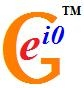# User blogs

Tag search results for: "alternative views"Hidden Dimensions Can Explain ‘Superluminal’ Neutrinos, and the Origin of Fermionic Mass (by Ray B. Munroe, Jr., Jonathan J. Dickau): Recent findings by OPERA indicate that neutrinos may travel faster than the speed of light. At face value, this implies that Einstein’s Theory of Relativity is either incorrect or requires an ad-hoc modification. But this result may be significant evidence for more dimensions than simply the four dimensions of Spacetime, which also has some interesting implications for particle physics. In addition; enough questions about the Standard Model Higgs boson have been raised by recent LHC data that it is wise to consider alternate mechanisms for fermionic mass. We explore how adding a family of ‘scalar fermions’ might address both of these issues. http://prespacetime.com/index.php/pst/article/view/296

The Errors of Statistical Hypotheses and Scientific Theories (Stephen P. Smith)

The process of error recognition is explored first in statistics, and then in science. The Type II error found in statistical hypothesis testing is found analogous to Karl Popper’s “logical probability” that is intended to measure the likelihood that a scientific theory can avoid its refutation. Nevertheless, Popper’s reliance on deductive thinking is found detracting from his demarcation that separates science and metaphysics. An improved critical logic for science is presented that permits error recognition more broadly: for induction by Popper’s falsification principle; but also for deduction and emotionality. The reality of induction creates a limitation for a science that has not accommodated a fuller menu of error recognition. The reality of induction places limits of what can be known from empiricism, and this has philosophical implications. http://prespacetime.com/index.php/pst/article/view/297

Kaluza-Klein Inflationary Universe in General Relativity (by Kishor S. Adhav)

Kaluza-Klein inflationary universe in general relativity has been studied. To obtain the deterministic model of the universe, it has been considered that the energy-momentum tensor of particles almost vanishes in the course of the expansion of the universe and thereby total energy-momentum tensor reduces to vacuum stress tensor. This assumption leads to (i) a ~ exp[Ht], where a is scale factor and H is Hubble constant. (ii) the effective potential V( ϕ) = constant, where ϕ is Higg’s field. It is observed that inflationary scenario is possible in Kaluza-Klein universe. http://prespacetime.com/index.php/pst/article/view/279

10 Reasons to Buy into Big Science (by Philip E. Gibbs)

When people hear the price tag for big science experiments like the Large Hadron Collider or the Hubble Space Telescope they wonder what the benefits are that justify the cost. I am not talking about projects with obvious potential benefits such as a fusion reactor. This is about pure science, why is it worth doing? In fact there are lots of reasons so here is my list of the top 10, starting with the least important. This article is based on viXra Log at http://blog.vixra.org. http://prespacetime.com/index.php/pst/article/view/301

· Aug 18 '12 ·The Nature of the Gravitational Field (by Antoine Acke): In this article we show that the introduction of g-information as the substance of the gravitational field and the hypothesis that the constitutive elements of this substance are informatons, permits to explain the - by experiments confirmed - nature of that field. http://prespacetime.com/index.php/pst/article/view/286

The Schrodinger-Equation Presentation of Any Oscillatory Classical Linear System that Is Homogeneous and Conservative (by Steven K. Kauffmann):

The time-dependent Schrodinger equation with time-independent Hamiltonian matrix is a homogeneous linear oscillatory system in canonical form. We investigate whether any classical system that itself is linear, homogeneous, oscillatory and conservative is guaranteed to linearly map into a Schrodinger equation. Such oscillatory classical systems can be analyzed into their normal modes, which are mutually independent, uncoupled simple harmonic oscillators, and the equation of motion of such a system linearly maps into a Schrodinger equation whose Hamiltonian matrix is diagonal, with h times the individual simple harmonic oscillator frequencies as its diagonal entries. Therefore if the coupling-strength matrix of such an oscillatory system is presented in symmetric, positive-definite form, the Hamiltonian matrix of the Schrodinger equation it maps into is h-bar times the square root of that coupling-strength matrix. We obtain a general expression for mapping this type of oscillatory classical equation of motion into a Schrodinger equation, and apply it to the real-valued classical Klein-Gordon equation and the source-free Maxwell equations, which results in relativistic Hamiltonian operators that are strictly compatible with the correspondence principle. Once such an oscillatory classical system has been mapped into a Schrodinger equation, it is automatically in canonical form, making second quantization of that Schrodinger equation a technically simple as well as a physically very interpretable way to quantize the original classical system. http://prespacetime.com/index.php/pst/article/view/294

Nonlinear Theory of Elementary Particles Part XIV: On Photon and Electron Structure (by Alexander G. Kyriakos)

In the present article is shown the equivalence of the description of particles as point-like in the framework of quantum theory and as non-point-like in the framework of the nonlinear theory of elementary particles (NTEP). It is shown that non-point electron explains many peculiarities of quantum theory with respect to the classical theory. It is shown that the non-point structure of the electron allows us to calculate the characteristics of the electron and, in particular, to prove the universality of the electron charge. http://prespacetime.com/index.php/pst/article/view/295

· Aug 17 '12 ·